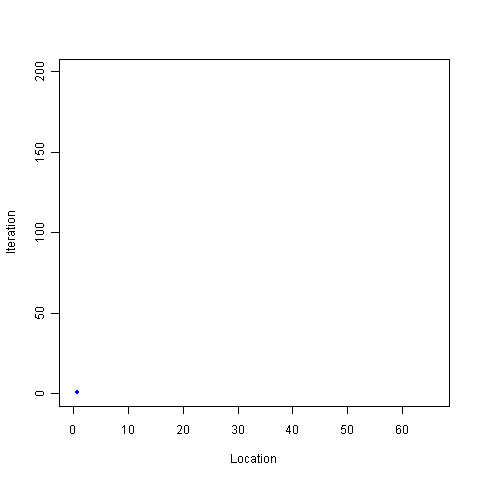[This article was first published on Probability and statistics blog » r, and kindly contributed to R-bloggers]. (You can report issue about the content on this page here)
Want to share your content on R-bloggers? click here if you have a blog, or here if you don't.Sample once from the Uniform(0,1) distribution. Call the resulting value$x$. Multiply this result by some constant$c$. Repeat the process, this time sampling from Uniform(0,$x*c$). What happens when the multiplier is 2? How big does the multiplier have to be to force divergence. Try it and see:

``` iters = 200 locations = rep(0,iters) top = 1 multiplier = 2 for(i in 1:iters) { locations[i] = runif(1,0,top)   top = locations[i] * multiplier }   windows() plot(locations[1:i],1:i,pch=20,col="blue",xlim=c(0,max(locations)),ylim=c(0,iters),xlab="Location",ylab="Iteration")   # Optional save as movie, not a good idea for more than a few hundred iterations. I warned you! # library("animation") # saveMovie(for (i in 1:iters) plot(locations[1:i],1:i,pch=20,col="blue",xlim=c(0,max(locations)),ylim=c(0,iters),xlab="Location",ylab="Iteration"),loop=1,interval=.1) ```